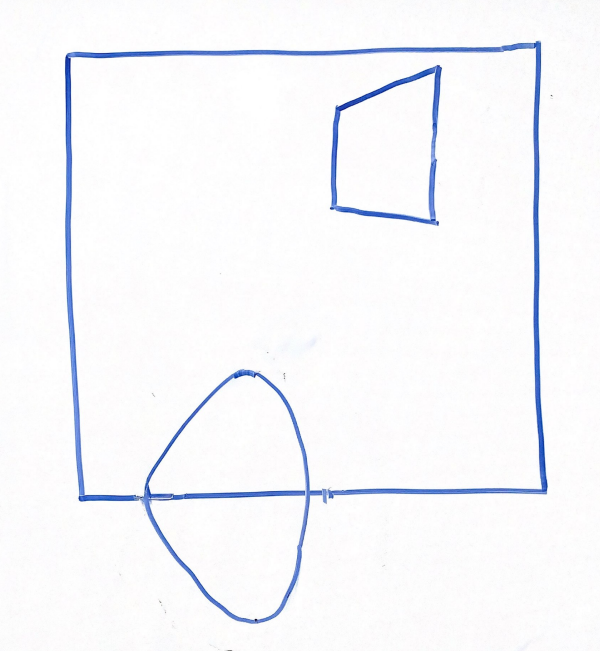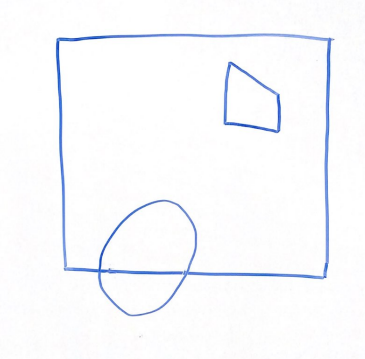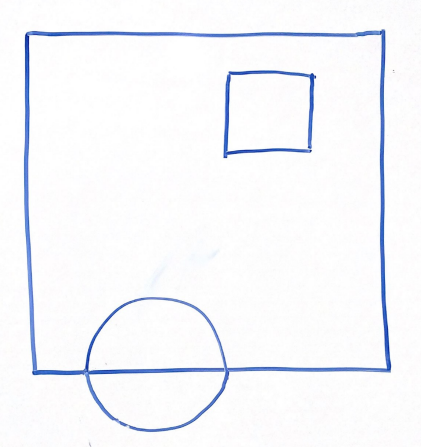# Project 5: Lights, Camera (Algo Answers)

You can find the handout for this project here.

## Projection Matrices

In lecture, we learned the two matrices that describe the camera: view and projection. In Ray, you learned how to generate a view matrix from a position, look, and up vector. In the Realtime projects, you will explore the concept of a projection matrix which covers the geometry of a view frustum!

### Visuals

For the above scene in a 2D frustum, which of the following options best represents the same scene after being projected into clip space via a perspective projection?

Option 1
Option 2Option 3
Option 4Option 3

Explanation:

Recall that when parallelizing the view frustum, the points closer to the edges will be moved more drastically than those near the midline.

Because of this, option 1 is incorrect, looking at the cube, the edges are equally distorted with respect to the midline.

Option 2 is incorrect because the vertices closer to the right side are stretched incorrectly outwards rather than towards the midline.

Option 4 is incorrect because no transformations occur to the shapes at all.

#### Scaling

Given a far plane distance of 100, height angle of 60 degrees, and a width angle of 90 degrees, what would the scaling matrix look like? Refer to this lecture for a reminder.

Explanation:

and , so the first diagonal entry is .

and , so the second diagonal entry is .

so the third diagonal entry is .

#### Unhinging

Given a near plane distance of 0.1 and a far plane distance of 100, what would the parallelization matrix look like? Refer to this lecture for a reminder.

Explanation:

, so and .

#### Converting to OpenGL Space

The matrix below is multiplied on the left side of the two matries above to convert to OpenGL's expected space. What are the x, y, and z limits in OpenGL's clip space and what is this doing to our previous projection to convert it to these limits?

Our current perspective transformations map to a space of . In order to map the axis to which is what OpenGL displays, we must first scale by -2 along z to both flip and fill a range of size 2, then shift by -1 along in order to center our space in the coordinate.

## Vertex Attributes

### Vertex Buffer Format

Using , , to represent a vertex i's position, , , for its color and , for its texture coordinates, fill in the following array for 2 vertices which have position, color, and texture coordinate attributes:

or

or

or

or

or

Explanation:

As long as each attribute is written successively and the permutation of the 3 attributes remains consistent between vertices, any order will do. It is up to you as the programmer to tell your VAO where each attribute is in the VBO and which layout location they reside at.

### Vertex Arrays

For each attribute above (position, color, and texture coordinate), please provide the stride and offset (pointer) in bytes using to reference size_of(GLfloat). (Assume , , , , , , , and are all of type GL_FLOAT)

StrideOffset
Position
Color
Texture Coordinates

Answer: In the same order as above, this is how you would specify the relative strides and offsets:

StrideOffset
Position
Color
Texture Coordinates

or

StrideOffset
Position
Color
Texture Coordinates

or

StrideOffset
Position
Color
Texture Coordinates

or

StrideOffset
Position
Color
Texture Coordinates

or

StrideOffset
Position
Color
Texture Coordinates

or

StrideOffset
Position
Color
Texture Coordinates

Explanation:

The stride will be for every attribute as the length of a vertex is the same regardless. The offset represents how many steps into the VBO that you will find the first element of a given attribute for the first vertex.

## Realtime Pipeline

### Rasterization

What does rasterization do? How does rasterization fit into the realtime pipeline in terms of inputs and outputs?

Rasterization is the process of determining which pixels should be covered based on the input vertex data. In the OpenGL pipeline, rasterization occurs between the vertex and fragment shaders. In GLSL terminology, the vertex shader output gl_Position is first clipped using the space and the resultant truncated geometries are used to check for covered pixels. These covered pixels are called "fragments" and are then processed in the fragment shader.

We can perform the same types of computation in both fragment and vertex shader. For this problem, compare the performance considerations associated with applying coordinate transforms in the vertex shader vs. the in fragment shader.

If we are drawing 1 triangle which covers exactly 50% of the pixels on an 1280 x 720 image, is it more efficient to compute an operation in the vertex shader or the fragment shader, why, and by how much so?

In addition, please give an example of a computation that must be performed in the fragment shader.

This triangle is composed of 3 vertices and covers pixels. As a result, the vertex shader will run times while the fragment shader will run times. Therefore it is times more efficient to perform the calculation in the vertex shader!

Some examples of fragment-only computations include diffuse dot products and specular dot products. Any computation that depends on the position at a given pixel in some way must be done in the fragment shader.

## Program Analysis

### Erroneous Code

Consider the following initializeGL pseudo-code:

	create VBO
bind VBO
fill VBO with data
unbind VBO
create VAO
bind VAO
set VAO attributes
unbind VAO


What would be the issue if a student tries calling glDrawArrays to draw their VAO in paintGL? How could you change this pseudo-code to remove the error?

The VAO currently is not linked to a VBO! The student would see a black screen :(. To fix the issue, moving the unbind VBO call to the end of this pseudocode will do the trick.

### Predict the Render

Given a VBO filled with data from an std::vector<GLfloat> of size 18, and VAO attribute code of:

glEnableVertexAttribArray(0);
glEnableVertexAttribArray(1);
glVertexAttribPointer(0, 3, GL_FLOAT, GL_FALSE, 6 * sizeof(GLfloat), nullptr);
glVertexAttribPointer(1, 3, GL_FLOAT, GL_FALSE, 6 * sizeof(GLfloat), reinterpret_cast<void *>(3 * sizeof(GLfloat)));


How many vertices and how many triangles are described in the VBO/VAO pair?

vertices and triangle.

Explanation:

In this case we see that each vertex is of size elements by looking at 6 * sizeof(GLfloat). Then given there are 18 elements, there are vertices. Each triangle is made out of triangles so there is triangle described by this VAO.

If the VAO attribute code was changed to:

glEnableVertexAttribArray(0);
glVertexAttribPointer(0, 3, GL_FLOAT, GL_FALSE, 3 * sizeof(GLfloat), nullptr);


How many vertices and how many triangles are now described in the VBO/VAO pair?

In this case we see that each vertex is of size elements by looking at 3 * sizeof(GLfloat). Then given there are 18 elements, there are vertices. Each triangle is made out of triangles so there are triangles described by this VAO.